# Analytics Calculations: Percentage of Total

Moderator, Lightspeed Staff Posts: 66 moderator
So now that we've learned how to start writing basic calculations, now that we have some the best practices and some of the more interesting functions to play with, let's try building some compound calculations.

Let's say I have a list of my sales by category for the year so far.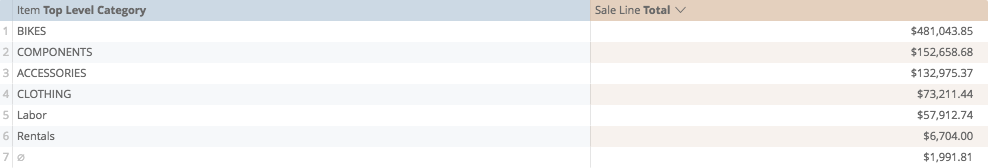But I want to determine the percentage of sales of each category.

To determine percentage, I need to divide each line by the total sales. I already have the value of each line, all that I am missing is the total.

So, I could create a new calculation just to determine the total. Let's use "Sum" that we just looked at in the Math Functions...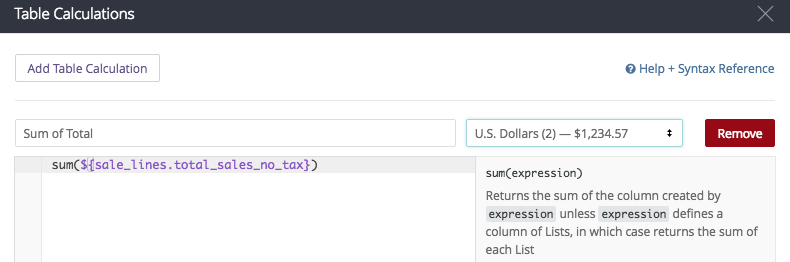So, my "Sum" calculation looks like:

sum(\${sale_lines.total_sales_no_tax})

and when I save it, it looks like...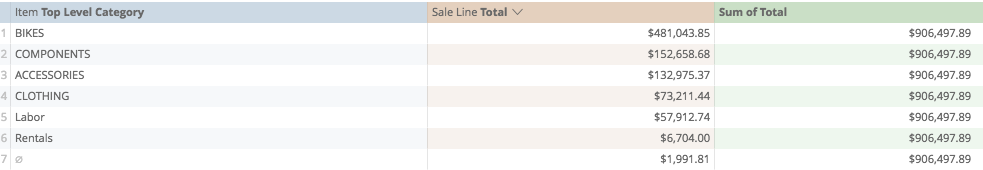...but of course I'm not finished. Now I need to divide the lines by the total.

So I'm going to go back to my calculations, and start a second table calculation...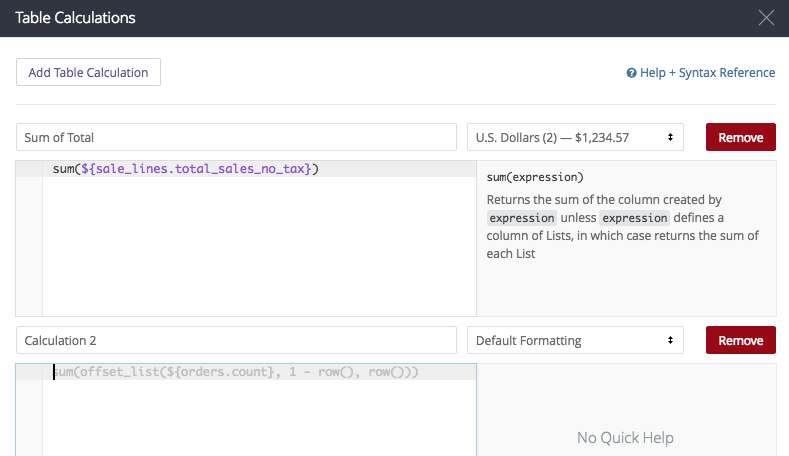and I'm going to start preparing my formula first, the line divided by something. I'm defining "something" here by a set of open/close brackets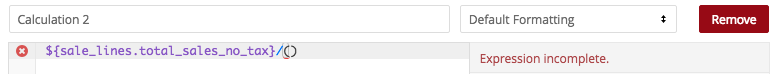Now we want to define what's inside those brackets. This is the easy part, we copy the formula from the first calculation...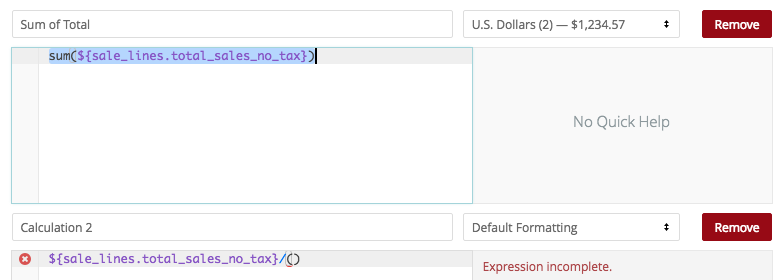...and then paste it between the brackets in the second calculation.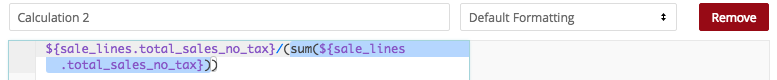So now, my calculation looks like...

\${sale_lines.total_sales_no_tax}/(sum(\${sale_lines.total_sales_no_tax}))

and if have prepared it properly, when I save...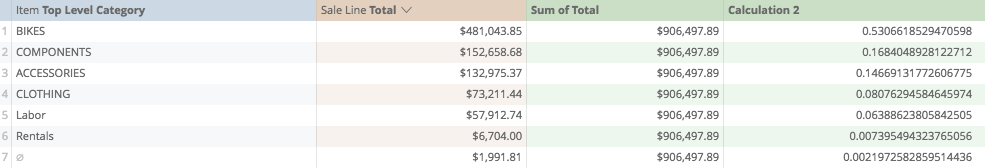Oh, now I wanted percentages, so I'm going to back to my table calculations, switch up the number formatting and give it a more meaningful title...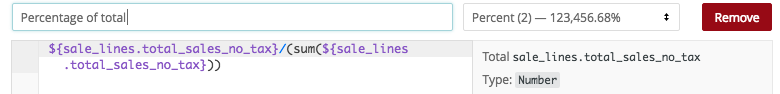and when I save it...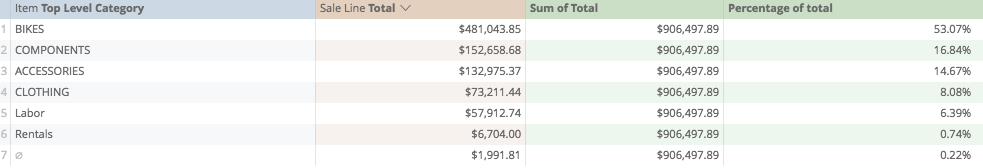finally, now that my second calculation looks good, I can remove my first calculation.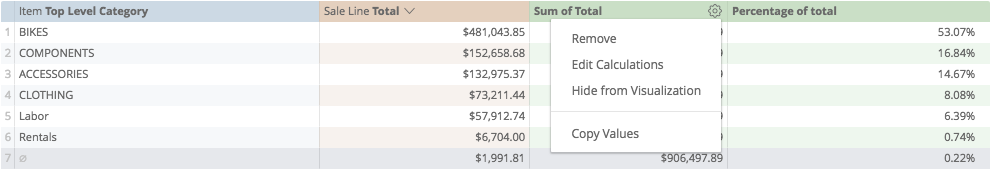Because the calculation for total is now implicit in the "Percentage of total", we don't need the first calculation taking up place on our table.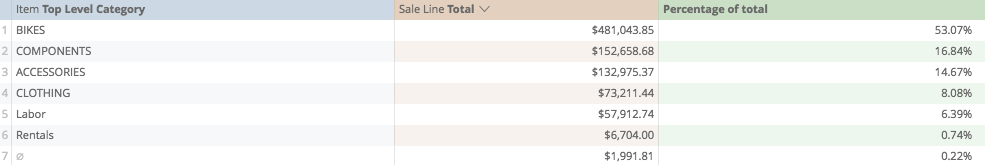and we finally have a clean report showing us the percentage each line contributes towards the total.

As an added bonus, because our calculation is not looking at the dimensions at all, we could immediately replace the dimension of "Top Level Category" with, say, Employee...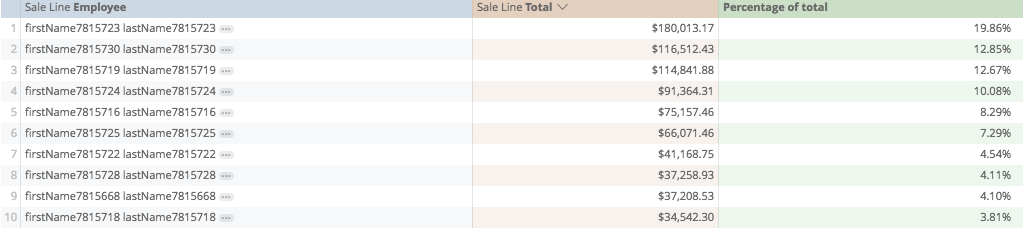or Multi-Store location...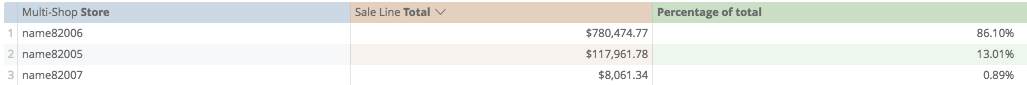or Customer...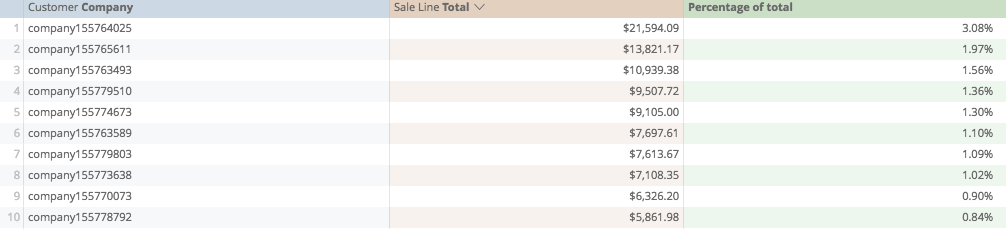And the possibilities go on...

Calculations Home
Joseph McKeown
Lightspeed Analytics and reporting consultant
Lightspeed HQ